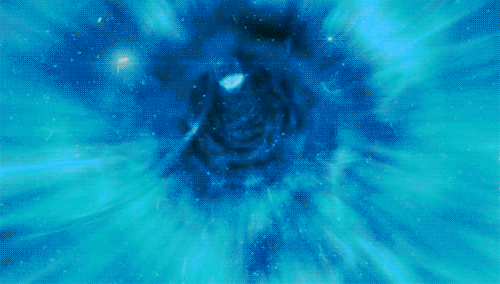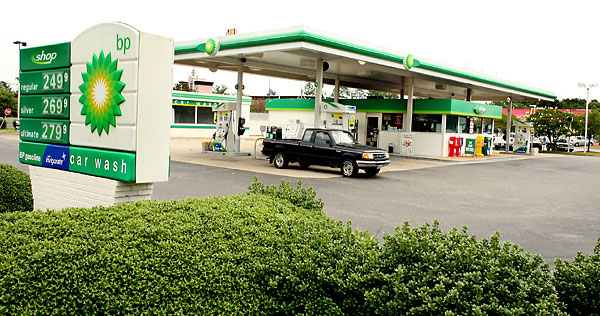Computer Science

# Simulation Techniques: Level 4 ChallengesChaos has emerged in a 1×1 field; The fabric of space will never be healed.

The limits of light have been replaced: All matter is teleported to a random place!

How far is the average point displaced?

This rule of space should not be disregarded: You might teleport to exactly where you started.

Hint: Don't set your bar low. Use Monte-Carlo.Consider a $100 \times 100$ grid. Suppose four points are randomly chosen on the grid. What is the probability that the convex hull of these four points is a quadrilateral?

For reference on Monte-Carlo, here is an excellent wiki.

##### Other approaches are definitely encouraged too.Some people buy their gas whenever their tank reaches empty. A smarter strategy (which we'll call "Wait long, buy low") is to wait for a good price, and then buy a lot of it. Suppose the price of fuel per gallon, $g$, on any given day follows a probability distribution $p_G(g)$.

To use the "Wait long, buy low" strategy, one buys gas once per month on the first day that the price is unlikely to be lower in a run of 30 days. If the price doesn't satisfy that condition in the first 29 days, then you buy gas on the 30th day, regardless of its price.

What is the average amount (in dollars) that you pay for gas per gallon in any given month?

Assumptions and Details

• For simplicity, let $p_G$ be the Gaussian $\displaystyle p_G(g) = \dfrac{1}{\sqrt{2\pi \sigma_G}} \exp\left[{-\frac{\left(g-\bar{g}\right)}{2\sigma_G^2}}\right]$ where $\bar{g}=\3.00$, and $\sigma_G = \0.25$.
• An event $E$ is unlikely if $p(E)<0.5$.
×